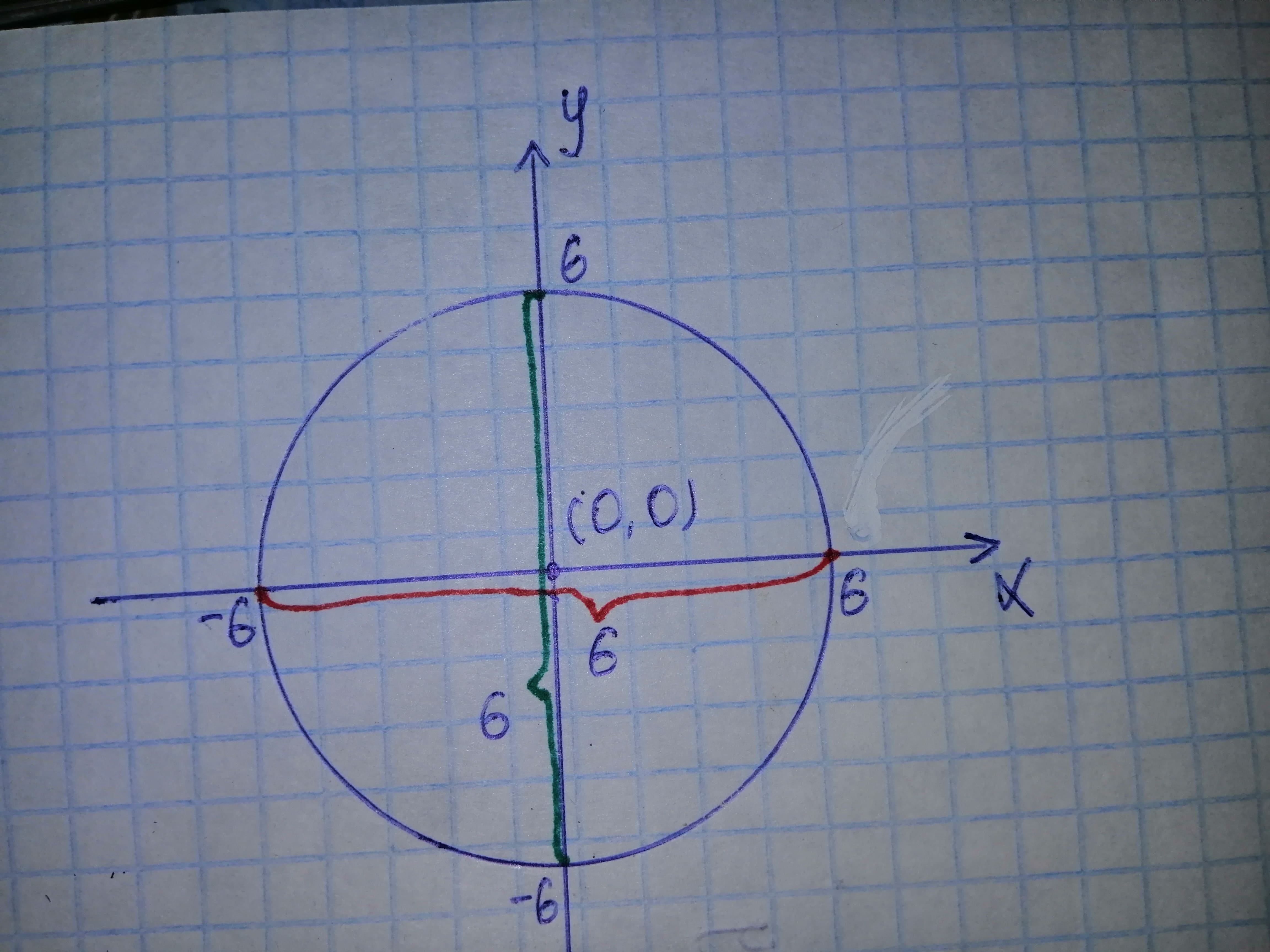# Find inequalities that describe the solid and state the coordinate system used. Position the solid on the coordinate system such that the inequalities are as simple as possible. "The solid that remains after a hole 1 inch in diameter is drilled through the center of a sphere 6 inches in diameter "pancha3 2020-11-02 Answered
Find inequalities that describe the solid and state the coordinate system used. Position the solid on the coordinate system such that the inequalities are as simple as possible. "The solid that remains after a hole 1 inch in diameter is drilled through the center of a sphere 6 inches in diameter "
You can still ask an expert for help

• Live experts 24/7
• Questions are typically answered in as fast as 30 minutes
• Personalized clear answers

Solve your problem for the price of one coffee

• Math expert for every subject
• Pay only if we can solve itSchulzD

Step 1
From the given hypothesis we clearly drawing a circle with centered at origin and with diameter is 6 inches:Step 2
The Coordinate systems are:
on x - axis
And also it passes through the centre (0, 0)
Therefore the inequalities are

We have step-by-step solutions for your answer!